Math Concepts
Algebra vs Calculus
52.7k views

 1 Introduction 2 Algebra vs Calculus 3 Linear Algebra vs Calculus 4 Linear algebra Vs Multivariable Calculus 5 Is Linear Algebra Harder Than Calculus? 6 About Cuemath 7 FAQs on Algebra vs Calculus 8 External references

23 October  2020

## Introduction

Algebra and Calculus both belong to different branches of mathematics and are closely related to each other. Applying basic algebraic formulas and equations, we can find solutions to many of our day-to-day problems.

Calculus is mostly applied in professional fields due to its capacity for finding solutions to many types of structures.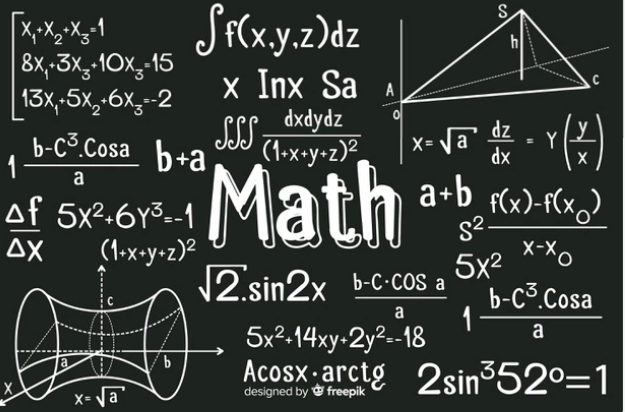### Algebra

Algebraic formulas and concepts are used in computing almost all mathematical problems. Algebra is indispensable in solving problems of arithmetic, trigonometry, and Calculus, etc. apart from its use in finding solutions to problems of other branches of science and technology.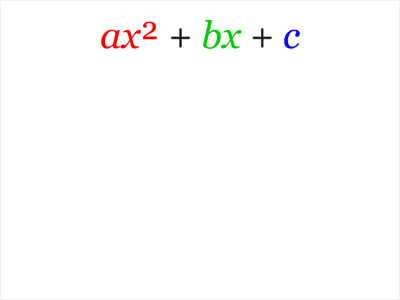Algebra is further branched as abstract algebra, linear algebra, universal algebra, elementary algebra, algebraic geometry, algebraic number theory, and algebraic systems, etc.

### Calculus

Calculus needs more brainwork than algebra to understand. It is the study of change in functions and their derivatives using a limiting process.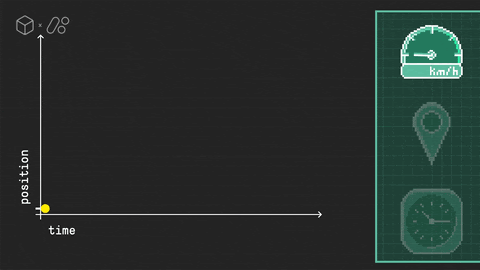It is the mathematical branch that uses small increments or decrements with particular reference to the rate of growth to arrive at solutions to a wide range of problems in science and technology. Calculus is further divided into

differential calculus and integral calculus.

If you ever want to read it again as many times as you want, here is a downloadable PDF to explore more.

## Algebra vs Calculus

Algebra and Calculus are closely related as much as one has to constantly use algebra while doing calculus. Being familiar with algebra, makes one feel comfortable with calculus. Algebra will let you grasp topics in calculus better and vice versa. But we can also do an analysis of algebra vs calculus

The following numbered list describes the broad topic “algebra vs calculus” and application difference between algebra and calculus.

1. Algebra focuses on solving equations whereas calculus is primarily focused on the rate of change of functions. The two main operations of calculus are differentiation (find the rate of change of a function) and integration (find the area under a curve of a function).
2. These two important operations are linked together by the fundamental theorem of calculus.
3. Algebra as in most mathematical disciplines is an important tool in calculus, and a good understanding of algebra and limits is fundamental to understanding calculus
4. Calculus deals with operations on functions and their derivatives whereas algebra deals with operations on variables and numbers.
5. Algebra assists in finding the slope of a line while calculus is for finding the slope of a curve.
6. Algebra helps in finding the direction of motion along a straight line whereas calculus does the same along any curve.
7. Algebra is used for finding the length of a line segment while calculus is used to find that of a piece of curve.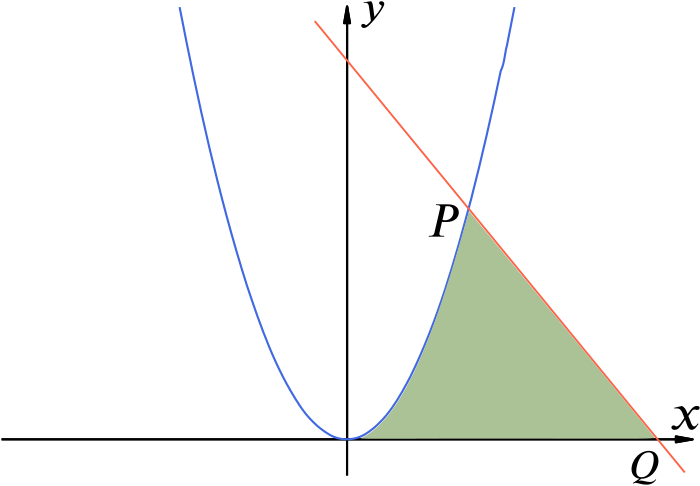To conclude, though there are many points for Algebra vs Calculus, Algebra and Calculus like everything in mathematics have a synergy and are used together in solving problems.

## Linear Algebra vs Calculus

Linear algebra is the study of the properties of vector spaces and matrices. Calculus and linear algebra are fundamental to virtually all of higher mathematics and its applications in the natural, social, and management sciences.

These topics, therefore, form the core of the basic requirements in mathematics both for mathematics majors and for students of science and engineering.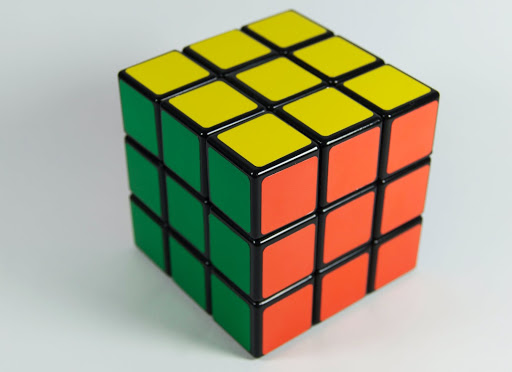The following points describe the broad topic “linear algebra vs calculus“ and the application difference between linear algebra and calculus.

1. The pure mechanics of Linear algebra are very basic, being far easier than anything of substance in Calculus. The difficulty is that linear algebra is mostly about understanding terms and definitions and determining the type of calculation and analysis needed to get the required result.
2. Linear algebra is easier than elementary calculus. Once the theorems in linear algebra are well understood most difficult questions can be answered.
3. This doesn't apply to calculus and computational questions in calculus could be very embarrassing even with a deep rigorous understanding of the materials.
4.  À heavily involved Calculus class brainstorming the application of derivatives may be considerably more difficult than an algebra class of Linear algebra course focused on computation.
5.  While the fundamentals of Linear algebra are easier to understand, the rules of Calculus can be confusing during their application.
6. Calculus can be used to predict the growth of plants, stresses on moving parts, stress points on bridges, high rise buildings, and other structures.The derivatives can take you from velocity to acceleration, but to visualize its practical application you need to learn physics and computer science, etc.

The concepts of linear algebra are extremely useful in physics, economics, social and natural sciences, and engineering.

## Linear algebra Vs Multivariable Calculus -

Linear algebra is called linear because it is the study of straight lines. It is the mathematics for solving systems modeled with multiple linear functions.

Many systems in nature can be described by multiple linear equations. Being easy to solve, every area of modern science contains models where equations are approximated to linear equations.

In Multivariable Calculus, we study functions of two or more independent variables e.g. z =f(x,y), p= f(x,y,z) etc. Multivariable Calculus expands on your knowledge of single variable calculus and applies to the 3D world.

In other words, we will be exploring the functions of two variables which are described in the three-dimensional coordinate system.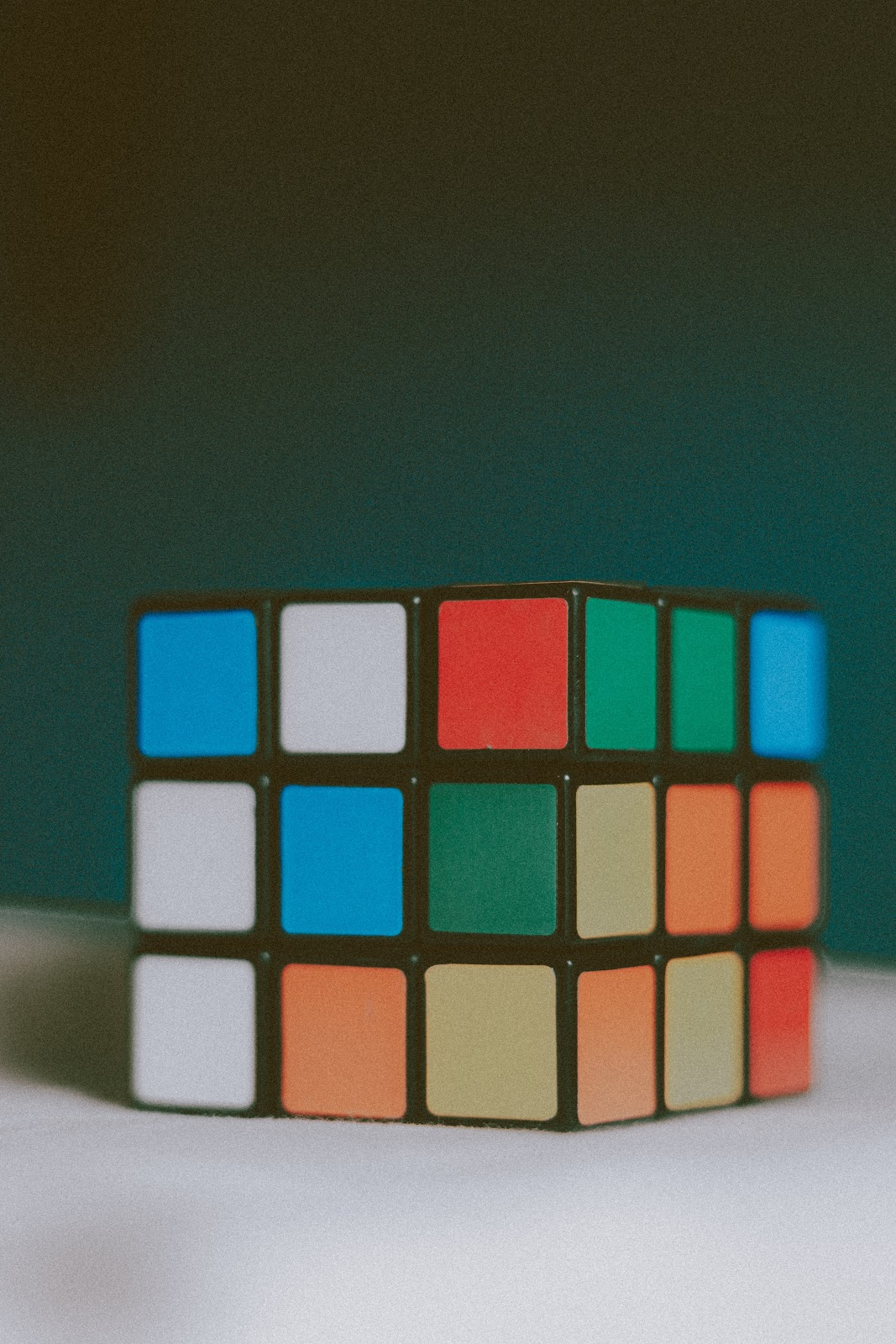To conclude, although llinear algebra vs multivariable calculus display different difficulty levels their interdependence and contributions to science and technology can't be ruled out.

## Is Linear Algebra Harder Than Calculus?

As the name goes by, linear algebra is the study of straight lines involving linear equations. Calculus is about understanding smoothly changing things involving derivatives, integrals, vectors, matrices, and parametric curves, etc.

So, is linear algebra harder than calculus?

The following mentions the level of difficulty of the question is linear algebra harder than calculus.

• Linear algebra needs less brain work than Calculus. Linear algebra is easier than elementary calculus. In Calculus, you can get by without understanding the intuition behind theorems and just memorizing algorithms, which won't work well in the case of linear algebra.
• By understanding the theorems in linear algebra, all questions can be solved. This doesn't apply to Calculus and computational questions can be very hard even with good knowledge of theory.
• You are not going to learn new technologies every day in linear algebra like that of Calculus. Newton's method, the volume of a cylinder, quotient rule, definition of a limit, and many more, hard to remember, are part of the calculus course.
•  Calculus 3 or Multivariable Calculus is the hardest mathematics course. Calculus is the hardest mathematics subject and only a small percentage of students reach Calculus in high school or anywhere else.
• Linear algebra is a part of abstract algebra in vector space. However, it is more concrete with matrices, hence less abstract and easier to understand.• Both Linear algebra and calculus involve determining length, area, and volume. As for determining length, Linear algebra deals with straight lines involving linear equations, whereas calculus may calculate the length of curved lines involving nonlinear equations with exponents which are harder to crack than linear ones.

Similarly considering area and volume, Linear algebra deals with areas of perfect circles and volumes of regularly shaped solids, while calculus is used to find enclosed areas with curved borders and volumes of irregularly shaped solids.

While the former uses simple quadratic equations, the latter uses equations with higher exponents which are certainly harder than the simple quadratic equations.

Having answered “is linear algebra harder than calculus”, the difficulty of a subject depends on the aptitude of the person for the subject. Hence a subject difficult for someone could be easy for some other. There will certainly be a difference of opinion. Therefore it is difficult to conclude  “is linear algebra harder than calculus?” most definitely.

## Summary

The debate over which is more difficult - algebra or calculus is a classic one. It will go on forever. However, let us summarise their broad similarities and differences here.

1. Algebra and Calculus though belong to different branches of math, they are inseparably related to each other. Looking into algebra vs calculus, applying basic algebraic formulas and equations, we can find solutions to many of our day-to-day problems.

Calculus is mostly applied in professional fields due to its capacity for finding solutions to many types of structures

2. Being familiar with algebra, makes one feel comfortable with calculus. Algebra will let you grasp topics in calculus better and vice versa. In fact, a good understanding of algebra helps one master calculus better.

Hence that must be kept in mind after learning about algebra vs calculus.

3. As for linear algebra vs calculus, let’s see the context with help of an example. Both linear algebra and calculus involve determining length, area, and volume.

As for determining length, Linear algebra deals with straight lines involving linear equations, whereas calculus may calculate the length of curved lines involving nonlinear equations with exponents. Which path a person chooses to reach to his answer depends upon his mathematical thinking.

4. As for answering, “Is linear algebra harder than calculus?”

Multivariable Calculus is considered the hardest mathematics course. Calculus is the hardest mathematics subject and only a small percentage of students reach Calculus in high school or anywhere else.

5. However, as with all other disciplines, the choice which is more difficult finally depends upon the interest and aptitude of the person pursuing it.

Written by Aarti Agarwal

Cuemath, a student-friendly mathematics platform, conducts regular Online Classes for academics and skill-development and their Mental Math App, on both iOS and Android, is a one-stop solution for kids to develop multiple skills. Understand the Cuemath Fee Structure and sign up for a free trial.

## Is algebra the same as calculus?

No. Though they are closely related, they both belong to different branches of mathematics. While calculus deals with operations on functions and their derivatives, algebra involves operations on numbers and variables.

## Can you learn calculus without studying algebra?

The answer again is no. Calculus builds on our knowledge of algebra and it’s a step ahead of algebra. It will be next to impossible to learn or understand calculus if one doesn't have a strong foundation in algebra.

## Why is algebra considered hard?

Algebra is different than most other branches of math in that it involves logical thinking about numbers. Most people tend to think of numbers in terms of arithmetic sense rather than algebraic sense. Hence, they find it difficult. Once, you learn to think in an algebraic sense you will find it a lot easy.

## What is the difference between algebra and calculus?

Algebra focuses on solving equations whereas calculus is primarily focused on the rate of change of functions. The two main operations of calculus are differentiation (find the rate of change of a function) and integration (find the area under a curve of a function).

1. These two important operations are linked together by the fundamental theorem of calculus.
2. Algebra as in most mathematical disciplines is an important tool in calculus, and a good understanding of algebra and limits is fundamental to understanding calculus
3. Calculus deals with operations on functions and their derivatives whereas algebra deals with operations on variables and numbers.
4. Algebra assists in finding the slope of a line while calculus is for finding the slope of a curve.
5. Algebra helps in finding the direction of motion along a straight line whereas calculus does the same along any curve.
6. Algebra is used for finding the length of a line segment while calculus is used to find that of a piece of curve.

Related Articles